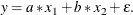Example 13.1 Nonnormal Error Estimation

This example illustrates the difference between GME-M and GME. One of the basic assumptions of OLS estimation is that the errors in the estimation are normally distributed. If this assumption is violated, the estimated parameters are biased. For GME-M, the story is similar. If the first moment of the distribution of the errors and a scale factor cannot be used to describe the distribution, then the parameter estimates from GME-MN are more biased. GME is much less sensitive to the underlying distribution of the errors than GME-M.

To illustrate this, data for the following model is simulated with three different error distributions:For the first simulation,is distributed normally, then a chi-squared distribution with six degrees of freedom is assumed for the second simulation, and finallyis assumed to have a Cauchy distribution in the third simulation.

In each of the three simulations, 100 samples of 10 observations each were simulated. The data for the model with the Cauchy error distribution is generated using the following DATA step code:

data one;
call streaminit(156789);
do by = 1 to 100;
do x2 = 1 to 10;
x1 = 10 * ranuni( 512);
y = x1 + 2*x2 + rand('cauchy');
output;
end;
end;
run;


The statements for the other distributions are identical except for the argument to the RAND() function.

The parameters to the model were estimated by using maximum entropy with the following programming statements:

proc entropy data=one gme outest=parm1;
model y = x1 x2;
by by;
run;


The estimation by using moment-constrained maximum entropy was performed by changing the GME option to GMEM. For comparison, the same model was estimated by using OLS with the following PROC REG statements:

proc reg data=one outest=parm3;
model y = x1 x2;
by by;
run;


The 100 estimations of the coefficient on variable x1 are then summarized for each of the three error distributions by using PROC UNIVARIATE, as follows:

proc univariate data=parm1;
var x1;
run;


The following table summarizes the results from the estimations. The true value for the coefficient on x1 is 1.0.

Estimation

Normal

Chi-Squared

Cauchy

Method

Mean

Std Deviation

Mean

Std Deviation

Mean

Std Deviation

GME

0.418

0.117

0.626

.330

0.818

3.36

GME-M

0.878

0.116

0.948

0.427

3.03

13.62

OLS

0.973

0.142

1.023

0.467

5.54

26.83

For normally distributed or nearly normally distributed data, moment-constrained maximum entropy is a good choice. For distributions not well described by a normal distribution, data-constrained maximum entropy is a good choice.